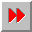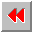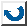EMA-XPS Online

LET*

```LET*
====

syntax: (let* ({<variable> |
(<variable> <value>) ...}) {<form> ...})

This function defines local variables, which can be
used in the <form>s. The start-value can be in every
form. If the start-value is not set, then NIL will
be used. LET* returns the value of the last form.
The difference between LET and LET* is: LET* defines
all variables parallel!

example:       >global         ; def. global-variable
123
>constant	; defined constant
123
>(let* (x (y 789))
(list x global constant y))
(NIL 123 123 789)

```EMA-XPS Online